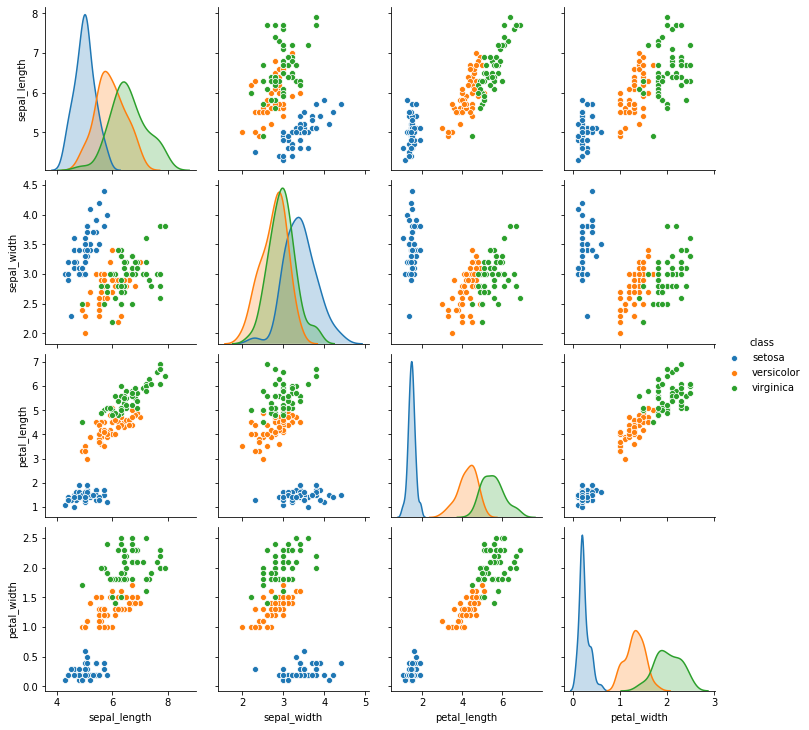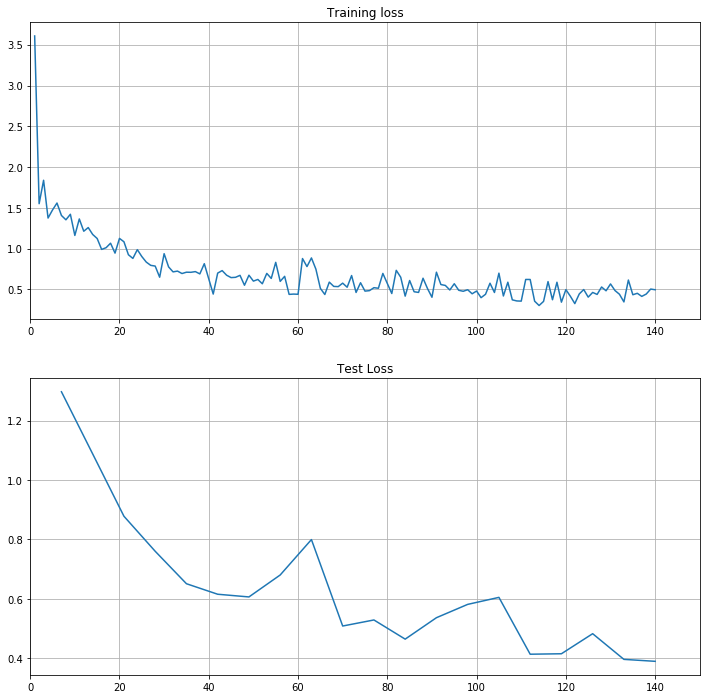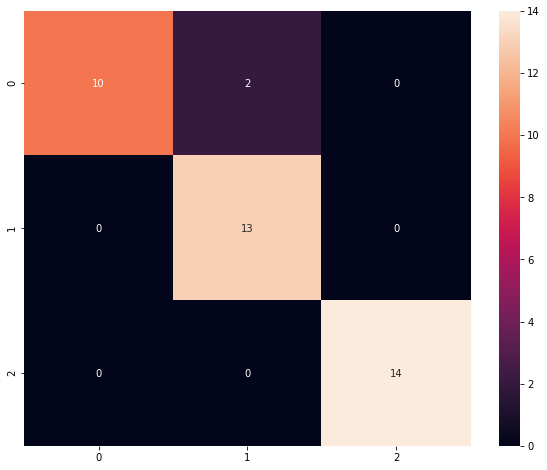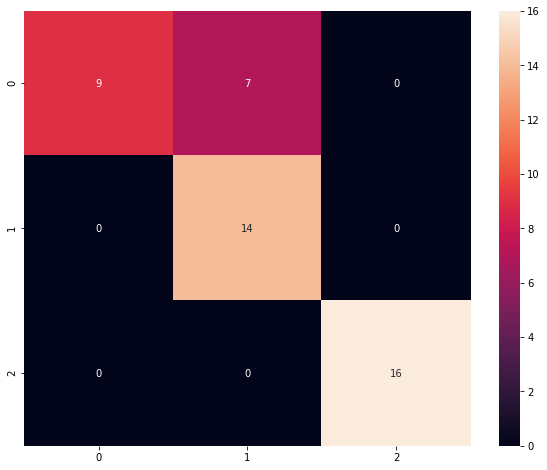# Hands-On: Logistic Regression with BigDL¶

### AI and Predictive Analytics in Data-Center Environments - http://dcai.bsc.es¶

Now that we know how to create a simple linear regression model both in bare bigDL and with PipelineML, let's move to the slightly more elaborate logistic regression. This example will help us to illustrate how networks with more than one layer are constructed in bigDL, as well as serve as further practice.

Because at the moment of writing this guide the RDD API is being deprecated and bare bigDL requires RDD manipulation we will use from now on the ML Pipeline API to train and test our models.

For this exercise we will use the well known Iris dataset, hosted at the UCI Machine Learning Repository. In order to pull the data directly from the repository you can use sc.addFile() (we can do this because the file is quite small, avoid doing this for large files) and then it can be loaded into a DataFrame with spark.read.format():

In :
from pyspark import SparkFiles
from pyspark.sql.types import StructType, StructField, FloatType, StringType
from pyspark import SparkContext
from bigdl.util.common import *
from pyspark.sql import SparkSession
# Create Spark Session
sc = SparkContext.getOrCreate(conf=create_spark_conf().setMaster("local").set("spark.driver.memory","10g"))
spark = SparkSession.builder.getOrCreate()

# Set a seed for RNG repeatability
seed = 2019

# Define te amount of features and classes for later use
num_features = 4
num_classes = 3

# Declare the DataFrame schema
iris_schema = StructType([
StructField('sepal_length', FloatType(), True),
StructField('sepal_width', FloatType(), True),
StructField('petal_length', FloatType(), True),
StructField('petal_width', FloatType(), True),
StructField('class', StringType(), True)])

# Have to add the format change to avoid an error
iris_raw = spark.read.option("timestampFormat", "yyyy/MM/dd HH:mm:ss ZZ") .format("csv")\
.schema(iris_schema)\
print('Number of rows: {}'.format(iris_raw.count()))
iris_raw.show(5)
iris_raw.printSchema()

Number of rows: 150
+------------+-----------+------------+-----------+-----------+
|sepal_length|sepal_width|petal_length|petal_width|      class|
+------------+-----------+------------+-----------+-----------+
|         5.1|        3.5|         1.4|        0.2|Iris-setosa|
|         4.9|        3.0|         1.4|        0.2|Iris-setosa|
|         4.7|        3.2|         1.3|        0.2|Iris-setosa|
|         4.6|        3.1|         1.5|        0.2|Iris-setosa|
|         5.0|        3.6|         1.4|        0.2|Iris-setosa|
+------------+-----------+------------+-----------+-----------+
only showing top 5 rows

root
|-- sepal_length: float (nullable = true)
|-- sepal_width: float (nullable = true)
|-- petal_length: float (nullable = true)
|-- petal_width: float (nullable = true)
|-- class: string (nullable = true)



Because the file we pulled from the UCI repository did not include the column headers we had to manually specify them by creating the DataFrame schema (following the dataset description provided by UCI) and passing it to spark.read.format.

You can see that all labels in columnclass are preceded by Iris-. This prefix does not add any kind of information, so although it is not strictly necessary let's get rid of it to reduce some clutter:

In :
import pyspark.sql.functions as f
iris_df = iris_raw.withColumn('class', f.split(f.col('class'), '-'))
iris_df.show(5)

+------------+-----------+------------+-----------+------+
|sepal_length|sepal_width|petal_length|petal_width| class|
+------------+-----------+------------+-----------+------+
|         5.1|        3.5|         1.4|        0.2|setosa|
|         4.9|        3.0|         1.4|        0.2|setosa|
|         4.7|        3.2|         1.3|        0.2|setosa|
|         4.6|        3.1|         1.5|        0.2|setosa|
|         5.0|        3.6|         1.4|        0.2|setosa|
+------------+-----------+------------+-----------+------+
only showing top 5 rows



## Initializing the BigDL Engine¶

Do not forget to start the BigDL engine before running any BigDL code:

In :
from bigdl.util.common import init_engine
init_engine()


Strings in a DataFrame column can be split with the split(column, separator) function, passing the corresponding columns and separator. split returns an array with all the tokens it finds.

## Exploring the data¶

Let's take a quick look to what the data looks like:

In :
import seaborn as sns
sns.pairplot(iris_df.toPandas(), hue="class");Out of the three possible classes, you can see that setosa stands out as it is particularly well separated from the other to, who appear more entangled in most scatter plots. We expect then our classifier to do a quite good job on distinugishing setosa and an OK one for the other versicolor and virginica.

## Preprocessing¶

We will seize the fact that we read the data directly into DataFrame format to make all the necessary data transformations using Spark ML and then take the DataFrame's internal RDD out when necessary.
The first step is to convert all categorical values to numerical. Clasifiers in BigDL only take float values both for their labels and features, so we need to turn the strings in the class columns into floats. The second step is to assemble all feature columns into a vector as we already saw in the previous notebook:

Important: BigDL uses 1-indexed values for classification labels (Due to its Torch heritage). This means that for a binary classification problem, it expects labels to take values 1.0 and 2.0, rather than 0.0 and 1.0. Providing the wrong labels will cause the optimizer to crash when trying to train the model

In :
from pyspark.ml.feature import StringIndexer, VectorAssembler
from pyspark.ml import  Pipeline

# String Indexer
indexer = StringIndexer(inputCol='class', outputCol='label')

# Vector Assembler
assembler = VectorAssembler(inputCols=iris_df.columns[:num_features], outputCol='features')

# Define a SparkML pipeline for the preprocessing:
iris_prepped = Pipeline(stages = [indexer, assembler])\
.fit(iris_df)\
.transform(iris_df)\
.withColumn('label', f.col('label')+1)

iris_prepped.show(5)
iris_prepped.printSchema()

+------------+-----------+------------+-----------+------+-----+--------------------+
|sepal_length|sepal_width|petal_length|petal_width| class|label|            features|
+------------+-----------+------------+-----------+------+-----+--------------------+
|         5.1|        3.5|         1.4|        0.2|setosa|  3.0|[5.09999990463256...|
|         4.9|        3.0|         1.4|        0.2|setosa|  3.0|[4.90000009536743...|
|         4.7|        3.2|         1.3|        0.2|setosa|  3.0|[4.69999980926513...|
|         4.6|        3.1|         1.5|        0.2|setosa|  3.0|[4.59999990463256...|
|         5.0|        3.6|         1.4|        0.2|setosa|  3.0|[5.0,3.5999999046...|
+------------+-----------+------------+-----------+------+-----+--------------------+
only showing top 5 rows

root
|-- sepal_length: float (nullable = true)
|-- sepal_width: float (nullable = true)
|-- petal_length: float (nullable = true)
|-- petal_width: float (nullable = true)
|-- class: string (nullable = true)
|-- label: double (nullable = false)
|-- features: vector (nullable = true)



We have used a Spark ML pipeline to build all preprocessing steps into a single instruction.

## Building the logistic regression model¶

Let's now define our logistic regression model using the BigDL modelling framework. In order to build a logistic regression we need two layers: a linear layer and a LogSoftMax activation layer. As we saw in the previous notebook BigDL offers two different APIs for defining models, the Sequential API and the Functional API. For illustration purposes we will define a model in each one of them:

### Logistic Regression model with the Sequential API¶

With more than one layer we need to create a container to put them in. The simplest flavour is the Sequential container, that simply stacks the layers in the order they were added to it:

In :
from bigdl.nn.layer import Sequential, Linear, LogSoftMax
lr_seq = Sequential()
print(lr_seq)

creating: createSequential
creating: createLinear
creating: createLogSoftMax
Sequential[bc5ceee5]{
[input -> (1) -> (2) -> output]
(1): Linear[d209acbc](4 -> 3)
(2): LogSoftMax
}


### Logistic Regression model with the Functional API¶

In the Functional API models are defined as if layers were directed edges of a graph connecting its input and output:

In :
from bigdl.nn.layer import Model
lin = Linear(num_features, num_classes)()
lsm = LogSoftMax()(lin)
lr_fun = Model(lin, lsm)
for l in lr_fun.layers:
print(l)

creating: createLinear
creating: createLogSoftMax
creating: createModel
Linear[df0d5a6f](4 -> 3)
LogSoftMax[1e3fc9d7]


To connect two layers simply pass the first layer as a parameter to the second one.

## Training the model in bare BigDL¶

### Preparing the data¶

As we already saw in the Linear Regression notebook, bigDL models require their input to be represented as a RDD of Sample:

Important: Remember that the BigDL team instructs to only use from_ndarray to build Samples out of Numpy arrays in python.

In :
from bigdl.util.common import Sample
import numpy as np
iris_rdd = iris_prepped.select(['features', 'label'])\
.rdd.map(lambda x : Sample.from_ndarray(np.array(x), np.array(x)))
iris_rdd.take(5)

Out:
[Sample: features: [JTensor: storage: [5.1 3.5 1.4 0.2], shape: , float], labels: [JTensor: storage: [3.], shape: , float],
Sample: features: [JTensor: storage: [4.9 3.  1.4 0.2], shape: , float], labels: [JTensor: storage: [3.], shape: , float],
Sample: features: [JTensor: storage: [4.7 3.2 1.3 0.2], shape: , float], labels: [JTensor: storage: [3.], shape: , float],
Sample: features: [JTensor: storage: [4.6 3.1 1.5 0.2], shape: , float], labels: [JTensor: storage: [3.], shape: , float],
Sample: features: [JTensor: storage: [5.  3.6 1.4 0.2], shape: , float], labels: [JTensor: storage: [3.], shape: , float]]

As usual, we split the data into training and testing datasets

In :
train_test_rate = 0.7

train_rdd, test_rdd = iris_rdd.randomSplit([train_test_rate, 1-train_test_rate], seed)
print("Train observations: {}".format(train_rdd.count()))
print("Test observations: {}".format(test_rdd.count()))

Train observations: 111
Test observations: 39


Once the layout of the network has been defined, we need to setup the optimizer that will fit the network to the training data, as well as the training hyperparameters:

### Training the model¶

Now that the data is ready we need to define the Optimizer and associated hyperparameters. When dealing for classification, the Negative Log Likelihood (NLL) is the optimization method of choice:

In :
from bigdl.optim.optimizer import *
from bigdl.nn.criterion import *
from bigdl.optim.optimizer import *

batch_size = 16
epochs = 20
learning_rate = 0.05

optimizer = Optimizer(
model=lr_seq,
training_rdd=train_rdd,
criterion=ClassNLLCriterion(),
end_trigger=MaxEpoch(epochs),
optim_method=SGD(learningrate=learning_rate),
batch_size=batch_size)

creating: createClassNLLCriterion
creating: createMaxEpoch
creating: createDefault
creating: createSGD
creating: createDistriOptimizer


Because we also want to visualize the training process, let's also define a validator and logging. Loss() defaults to ClassNLLCriterion so we do not need to specify it for this case:

In :
# Define a validator for the Optimizer
optimizer.set_validation(
batch_size=batch_size,
val_rdd=test_rdd,
trigger=EveryEpoch(),
val_method=[Loss()]
)

# Define the Logs and pass them to the Optimizer
import datetime as dt
log_dir = '/tmp/bigdl_summaries'
app_name = 'iris-logreg-' + dt.datetime.now().strftime("%Y%m%d-%H%M%S")

# Create the train and validation summaries
train_summary = TrainSummary(log_dir=log_dir, app_name=app_name)
val_summary = ValidationSummary(log_dir=log_dir, app_name=app_name)

# Pass them to the optimizer
optimizer.set_train_summary(train_summary)
optimizer.set_val_summary(val_summary)
print("Logs saved to: {}/{}".format(log_dir, app_name))

creating: createEveryEpoch
creating: createClassNLLCriterion
creating: createLoss
creating: createTrainSummary
creating: createValidationSummary
Logs saved to: /tmp/bigdl_summaries/iris-logreg-20200204-165806


And finally train the linear regression model:

In :
optimizer.optimize();


### Validating the model¶

In order to validate the model, we can take a look at the training and validation logs:

In :
import matplotlib.pyplot as plt

plt.figure(figsize = (12,12))
plt.subplot(2,1,1)
plt.plot(loss_train[:,0],loss_train[:,1],label='Training loss')
plt.xlim(0,loss_train.shape+10)
plt.grid(True)
plt.title("Training loss")

plt.subplot(2,1,2)
plt.plot(loss_test[:,0],loss_test[:,1],label='Test loss')
plt.xlim(0,loss_train.shape+10)
plt.title("Test Loss")
plt.grid(True)And also evaluate the trained model against the test data:

In :
test_results = lr_seq.evaluate(test_rdd, batch_size, [Loss()])
print(test_results)

creating: createClassNLLCriterion
creating: createLoss
Evaluated result: 0.3898795545101166, total_num: 3, method: Loss


As usual, you can access the model coefficients using get_weights() on the model:

In :
print("Coefficients:\n{}".format(lr_seq.get_weights()))
print("Intercept:\n{}".format(lr_seq.get_weights()))

Coefficients:
[[ 0.08006627 -0.0890116   0.15397008 -0.11890944]
[-0.33158302 -0.2756649   0.591689    0.9279306 ]
[ 0.3536049   0.67081136 -0.97251445 -0.7639799 ]]
Intercept:
[ 0.2991739  -0.09824922  0.28697506]


A particularly effective way to visualize classifier performance is to use a confusion matrix. For that purpose we will use the utilities provided by sklearn:

In :
from sklearn.metrics import confusion_matrix
from sklearn.metrics import accuracy_score
import pandas as pd

# Remember that labels are 1-indexed in BigDL:
y_pred = np.array(lr_seq.predict_class(test_rdd).collect())-1
y_label = np.array([s.label.to_ndarray() - 1 for s in test_rdd.collect()])

acc = accuracy_score(y_label, y_pred)
print("The prediction accuracy is %.2f%%"%(acc*100))

cm = confusion_matrix(y_label, y_pred)
df_cm = pd.DataFrame(cm)
plt.figure(figsize = (10,8))
sns.heatmap(df_cm, annot=True,fmt='d');

The prediction accuracy is 94.87%## Training a model with ML Pipeline¶

### Preparing the data¶

We already transformed the data into the appropriate DataFrame format in the Preprocessing section, so we only need to split it into training and validation:

In :
train_df, test_df = iris_prepped.randomSplit([train_test_rate, 1-train_test_rate], seed)
print("Train observations: {}".format(train_df.count()))
print("Test observations: {}".format(test_df.count()))

Train observations: 104
Test observations: 46


Let's now define the logistic regression model. This model is composed of two layers, a linear layer and a LogSoftMax layer. In order to be able to put the layers together we will create a container### Training the model¶

For classification tasks instead of using DLEstimator we need to use DLClassifier to wrap the BigDL model. Recall that the DLEstimator and DLClassifier constructor does not takes values for the batch size, stopping trigger or learning rate, so we need to specify them afterwards. We will use the Functional model we already created:

In :
from bigdl.dlframes.dl_classifier import DLClassifier
lr_ml = DLClassifier(lr_fun, ClassNLLCriterion(), feature_size=[num_features])\
.setBatchSize(batch_size)\
.setMaxEpoch(epochs)\
.setLearningRate(learning_rate)

creating: createClassNLLCriterion
creating: createDLClassifier


To train the model, simply call fit() passing it the training data:

In :
lr_model = lr_ml.fit(train_df)


### Validating the model¶

In :
from pyspark.ml.evaluation import MulticlassClassificationEvaluator
evaluator=MulticlassClassificationEvaluator(predictionCol='prediction')
pred_test = lr_model.transform(test_df)\
.select('label', 'prediction')\
.withColumn('label', f.col('label')-1)\
.withColumn('prediction', f.col('prediction')-1)
acc = evaluator.evaluate(pred_test, {evaluator.metricName: "accuracy"})
print("The prediction accuracy is %.2f%%"%(acc*100))

The prediction accuracy is 84.78%

In :
# Remember that labels are 1-indexed in BigDL:
cm = confusion_matrix(pred_test.select('label').collect(), pred_test.select('prediction').collect())
df_cm = pd.DataFrame(cm)
plt.figure(figsize = (10,8))
sns.heatmap(df_cm, annot=True,fmt='d');